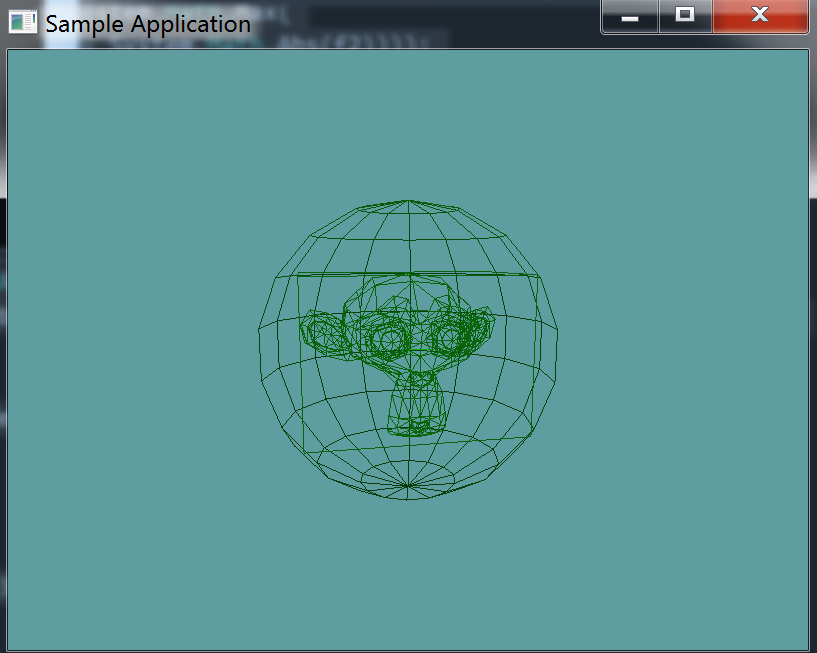# Sphere

In the last section we built an AABB around out model, this actually gives us everything we need to build a bounding sphere.

The center of the sphere is the same as the center of the AABB.

The radius of the sphere is either the distance between the center of the sphere and the min point or the max point. Take the length of both, and use the larger one.

## Creating the sphere

protected Sphere containerSphere = null;


Now, in the constructor, after you have created the AABB, make the sphere object. Follow the instructions in the introduction paragraph.

## Debug Render

Now, let's add a public getter for the sphere:

public Sphere BoundingSphere {
get {
return containerSphere;
}
}


And modify the debug-render method to draw this box:

public void DebugRender() {
containerAABB.Render();
containerSphere.Render();
foreach (Triangle trianlge in collisionMesh) {
trianlge.Render();
}
}


## Unit Test

You can Download the samples for this chapter to see if your result looks like the unit test.

The constructor only errors out if the bounding sphere is off. Otherwise the rest of the test is visual only. You should see susane in the testusing OpenTK.Graphics.OpenGL;
using Math_Implementation;
using CollisionDetectionSelector.Primitives;
using CollisionDetectionSelector;

namespace CollisionDetectionSelector.Samples {
class OBJDebugTrianglesSphere : Application {

public override void Intialize(int width, int height) {
GL.Enable(EnableCap.DepthTest);
GL.Enable(EnableCap.CullFace);
GL.Enable(EnableCap.Lighting);
GL.Enable(EnableCap.Light0);

GL.PolygonMode(MaterialFace.FrontAndBack, PolygonMode.Line);

GL.Light(LightName.Light0, LightParameter.Position, new float[] { 0.0f, 0.5f, 0.5f, 0.0f });
GL.Light(LightName.Light0, LightParameter.Ambient, new float[] { 0f, 1f, 0f, 1f });
GL.Light(LightName.Light0, LightParameter.Diffuse, new float[] { 0f, 1f, 0f, 1f });
GL.Light(LightName.Light0, LightParameter.Specular, new float[] { 1f, 1f, 1f, 1f });

if (obj.NumCollisionTriangles != 968) {
LogError("Suzanne triangle count expected at 968, got: " + obj.NumCollisionTriangles);
}
AABB test = new AABB(new Point(-1.367188f, -0.984375f, -0.851562f), new Point(1.367188f, 0.984375f, 0.851562f));
if (!AlmostEqual(test, obj.BoundingBox)) {
LogError("Expected bounting box:\n" + test.ToString() + "\n Got bounding box: \n" + obj.BoundingBox.ToString());
}

}

if (!AlmostEqual(obj.BoundingSphere.Position, new Point(0f, 0f, 0f))) {
LogError("Expected bounding sphere radius to be: (0, 0, 0), got: " + obj.BoundingSphere.Position.ToString());
}
}

private bool AlmostEqual(float f1, float f2) {
return (System.Math.Abs(f1 - f2) <= 0.00001f *
System.Math.Max(1.0f, System.Math.Max(
System.Math.Abs(f1), System.Math.Abs(f2))));
}

private bool AlmostEqual(Point p1, Point p2) {
return AlmostEqual(p1.X, p2.X) && AlmostEqual(p1.Y, p2.Y) && AlmostEqual(p1.Z, p2.Z);
}

private bool AlmostEqual(AABB aabb1, AABB aabb2) {
return AlmostEqual(aabb1.Min, aabb2.Min) && AlmostEqual(aabb1.Max, aabb2.Max);
}

public override void Render() {
base.Render();
DrawOrigin();

GL.PushMatrix();
GL.Scale(3.0f, 3.0f, 3.0f);
obj.DebugRender();
GL.PopMatrix();
}
}
}